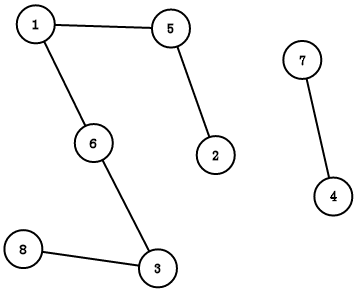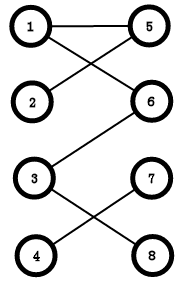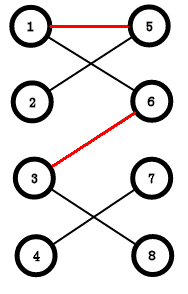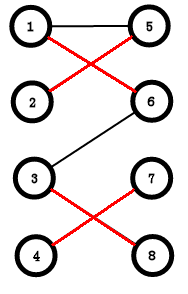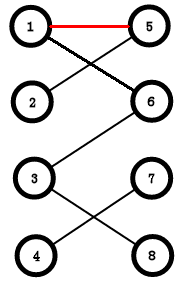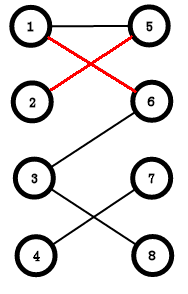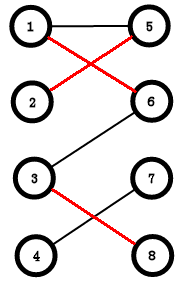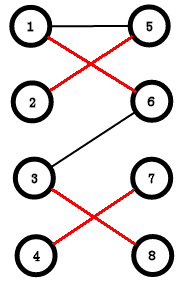vis//标记
a[i][j]//邻接矩阵标记i到j有一条边
bool dfs(int u) {
for(int i = head[u]; ~i; i = e[i].nx) {
int v = e[i].v;
if(!vis[v]) {
vis[v] = 1;
return 1;
}
}
}
return 0;
}

bool dfs(int u) {
for(int v = 1; v <= m; ++ v) {
if (!vis[v] && a[u][v]) {
vis[v] = 1;
return 1;
}
}
}
return 0;
}

//我们只要搜一侧就可以了
//如果这边的点多，那一定能遍历到另一边的所有点(如果有连边的话)
//如果这边的少，那最大匹配一定<=这边点的个数
for(int i = 1; i <= n; ++ i) {
memset(vis,0,sizeof(vis));
if(dfs(i)) ans ++;
}

König定理最大匹配 = 最小顶点覆盖

some例题：